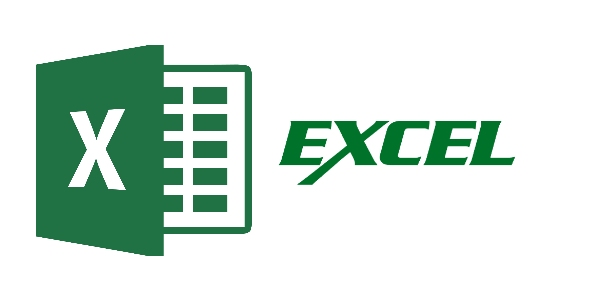32 Questions | Total Attempts: 134SettingsRelated Topics
• 1.
Once you hide a row, it remains hidden as long as the workbook is active.
• A.

True

• B.

False

• 2.
When a worksheet is printed, the grid lines that surround the cells are printed by default.
• A.

True

• B.

False

• 3.
A(n) ________ function is conditional.
• 4.
You can rotate cell contents in Excel so that they appear at any angle or orientation.
• A.

True

• B.

False

• 5.
After you merge a range into a single cell, you can't realign its content.
• A.

True

• B.

False

• 6.
In "SUM(number1 [,number2 ,number3 ...])" number 1, number 2, and number 3 may be numbers or __________.
• A.

Letters

• B.

Cell references

• C.

Symbols

• D.

Operators

• 7.
Formatting changes only the appearance of data- it does not affect the data itself.
• A.

True

• B.

False

• 8.
To enter a mixed reference, type \$ after either the row or column reference.
• A.

True

• B.

False

• 9.
All functions have arguments.
• A.

True

• B.

False

• 10.
If a formula contains several functions, Excel starts with the outermost function and then moves inward.
• A.

True

• B.

False

• 11.
As you begin to type a function name within a formula into a cell, a list of functions that begin with the letters you typed appears.
• A.

True

• B.

False

• 12.
FUNCTION(argument1,argument2, ...) is ________.
• A.

The syntax of writing optional arguments.

• B.

An example of a formula.

• C.

The general syntax of all functions.

• D.

The general syntax of all formulas.

• 13.
In the following formula: =IF(A1="YES","DONE","RESTART"), what happens if A1="NO"?
• A.

The formula returns the text "RESTART"

• B.

The formula returns the text "DONE"

• C.

The formula returns the text "NO"

• D.

The formula returns the text "NO, RESTART"

• 14.
The ____ button is equivalent to using the sum button.
• A.

COUNT

• B.

• C.

AutoSum

• D.

• 15.
The numbers, text, or cell references used by the function to return a value are
• A.

Expressions

• B.

Arguments

• C.

Data

• D.

Values

• 16.
In "SUM(number1 [,number2 ,number3 ...])", number 2 and number 3 are ________
• 17.
What uses vertical rectangles to compare values across categories.
• 18.
Excel has a built in format to display 4 digit year values, so you do not need to create on.
• A.

True

• B.

False

• 19.
What displays values in different categories; column chart on its side.
• 20.
What is a range of values that is plotted as a single unit on the chart.
• 21.
This compares relative values of different categories to the whole; similar to the pie chart except that it can display multiple sets of data.
• 22.
This compares a collection of values from several different data sets.
• 23.
Pie charts are most effective with ___________ or fewer slices.
• 24.
This is a collection of one or more data series.
• 25.
This compares three sets of values in a 3D chart.